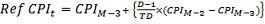### Issuers / Bond Info

Source:
Bureau of Trade and Economic Indices, Ministry of Commerce - http://www.price.moc.go.th.

Remark:
1. Reference CPI is calculated using the CPI data obtained from Bureau of Trade and Economic Indices, Ministry of Commerce - http://www.price.moc.go.th.
2. Reference CPI from April-2010 to February-2013 is calculated by CPI (Base B.E. 2550).
3. Reference CPI from March-2013 to February-2017 is calculated by CPI (Base B.E. 2554).
4. Reference CPI from March-2017 to February-2021 is calculated by CPI (Base B.E. 2558).
5. Reference CPI starting from March-2021 is calculated by CPI (Base B.E. 2562).
6. The following formula is used for deriving the Reference CPI on Day t.Remark:
1. Index Ratio from July-2011 to February-2013 is calculated by Reference CPI (Base B.E. 2550).
2. Index Ratio from March-2013 to February-2017 is calculated by Reference CPI (Base B.E. 2554).
3. Index Ratio from March-2017 to February-2021 is calculated by Reference CPI (Base B.E. 2558).
4. Index Ratio starting from March-2021 is calculated by Reference CPI (Base B.E. 2562).